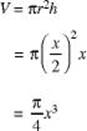﻿ ﻿Answers and Explanations - Three-Dimensional Geometry - Geometry and Measurement - REVIEW OF MAJOR TOPICS - SAT SUBJECT TEST MATH LEVEL 2

## PART 2## REVIEW OF MAJOR TOPICS## CHAPTER 2 Geometry and Measurement

###2.2 Three-Dimensional GeometrySurface Area and Volume

1. (C) The volume of the cube is x3. The radius of the cylinder is, and its height is x . Substitute these into the formula for the volume of a cylinder:2. (C) V = πr2h, and total area = 2πr2 + 2πrh . Setting these two equal yields rh = 2r + 2h . Therefore,. Since h must be positive, the smallest integer value of r is 3.

3. * (D) The length of the longest segment isCoordinates in Three Dimensions

1. (D) The square of the distance between P and Q is 9, so

(x – 3)2 + (–1 – (–3))2 + (–1 – 1)2 = 9, or (x – 3)2 = 1.

Therefore, x – 3 = ±1, so x = 2 or 4.

2. (D) Since the y -coordinate is zero, the point must lie in the xz plane.

3. * (C) The line 3x + 2y = 7 has x -interceptand y -intercept. The part of this line that lies in the first quadrant forms a triangle with the coordinate axes. Rotating this triangle about the x -axis produces a cone with radiusand height. The volume of this cone is.

﻿